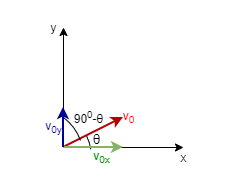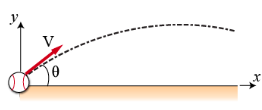# Problem: During a baseball game, a baseball is struck at ground level by a batter. The ball leaves the baseball bat with an initial velocity vo = 47 m/s at an angle θ = 21° above horizontal. Let the origin of the Cartesian coordinate system be the ball's position the instant it leaves the bat. Air resistance may be ignored throughout this problemExpress the magnitude of the ball's initial horizontal velocity v0x interms of v0 and θ

###### FREE Expert Solution

Let's visualize the motion as follows:87% (337 ratings)###### Problem Details

During a baseball game, a baseball is struck at ground level by a batter. The ball leaves the baseball bat with an initial velocity vo = 47 m/s at an angle θ = 21° above horizontal. Let the origin of the Cartesian coordinate system be the ball's position the instant it leaves the bat. Air resistance may be ignored throughout this problemExpress the magnitude of the ball's initial horizontal velocity v0x interms of vand θ